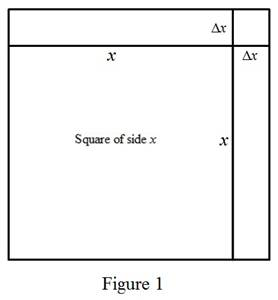# The value of A ′ ( 15 ) .### Single Variable Calculus: Concepts...

4th Edition
James Stewart
Publisher: Cengage Learning
ISBN: 9781337687805### Single Variable Calculus: Concepts...

4th Edition
James Stewart
Publisher: Cengage Learning
ISBN: 9781337687805

#### Solutions

Chapter 3.8, Problem 11E

(a)

To determine

## To find: The value of A′(15).

Expert Solution

The value of A(15) is 30mm2_.

### Explanation of Solution

Given:

The company makes square wafers of silicon of area of the form A(x)=x2.

Calculation:

Differentiate the area of the square wafer respect to x.

A(x)=x2

A'(x)=2x

Substitute 15 for x in the above equation and find A(15) as,

A'(15)=2(15)=30mm2

Therefore, the rate at which the area will increase with respect to change in its side length, if the side length is 15 mm will be 30mm2_.

(b)

To determine

Expert Solution

### Explanation of Solution

Draw the square with side length, x as shown in Figure (1).The perimeter of the square is P(x)=4x.

From the figure 1, the change in length Δx is very small.

The first derivative of A(x) is,

A'(x)=2x=12(4x)=12P(x)

Thus, the change in area of the square is approximately half of its perimeter which is half of the 4 sides times.

The expression for the shaded area (change in area) as below.

ΔA=2x(Δx)+(Δx)2

The change in length Δx is small, and change in area ΔA is approximately equal to 2x(Δx).

ΔA=2x(Δx)

The term ΔAΔx is approximately equal to 2x.

ΔAΔx=2x

ΔAΔx=12P(x)

Thus the rate of change of the area of a square with respect to the change in its side length is equal to half its perimeter is ΔAΔx=12P(x).

### Have a homework question?

Subscribe to bartleby learn! Ask subject matter experts 30 homework questions each month. Plus, you’ll have access to millions of step-by-step textbook answers!